Function Repository Resource:

# MixedEulerianGraphQ

Test if a strongly connected mixed graph is Eulerian or unicursal

Contributed by: Peter Cullen Burbery
 ResourceFunction["MixedEulerianGraphQ"][g] yields True if the strongly connected mixed graph g is Eulerian or unicursal, and False otherwise.

## Details

Eulerian graphs have applications in arc routing and operations research.
A mixed graph that is strongly connected is Eulerian, or unicursal, if and only if the graph is even (meaning all nodes/vertices are connected to an even number of other nodes/vertices and have an even degree) and the graph satisfied the balanced set condition, which states that for any subset of vertices/nodes, the difference between the number of arcs entering and the number of arcs leaving is less than or equal to the number of edges incident with the vertices/nodes.

## Examples

### Basic Examples (2)

Test if a mixed graph is Eulerian:

 In:=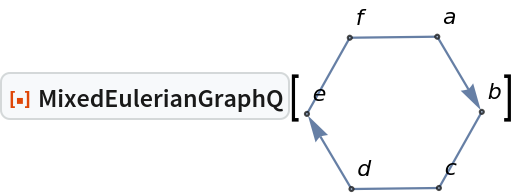Out=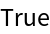Although this graph is even, the graph violates the balanced set condition and is therefore not Eulerian:

 In:=Out=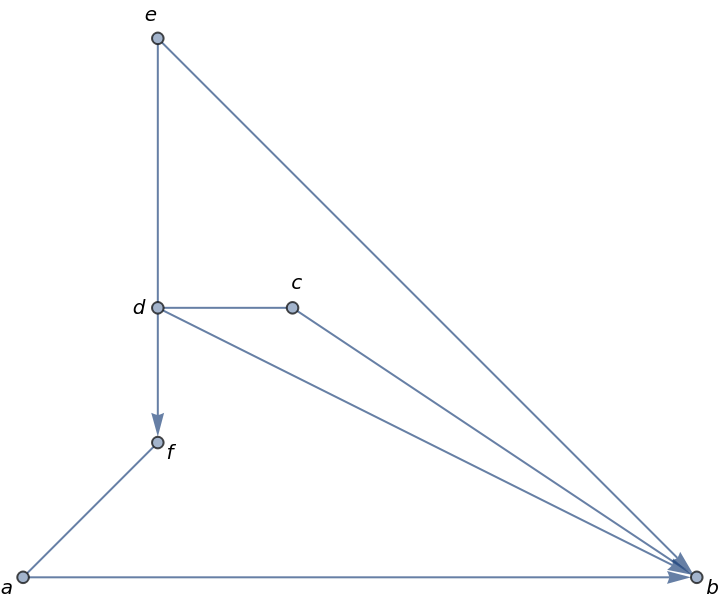In:=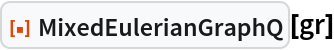Out=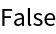### Possible Issues (1)

A graph that is beyond a limit of around 14 nodes can take an unreasonable amount of time because the number n of subsets grows exponentially according to O(2n):

 In:=Out=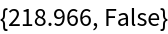Peter Burbery

## Version History

• 1.0.0 – 23 August 2022

## Author Notes

I wrote this function because EulerianGraphQ does not work on mixed graphs.